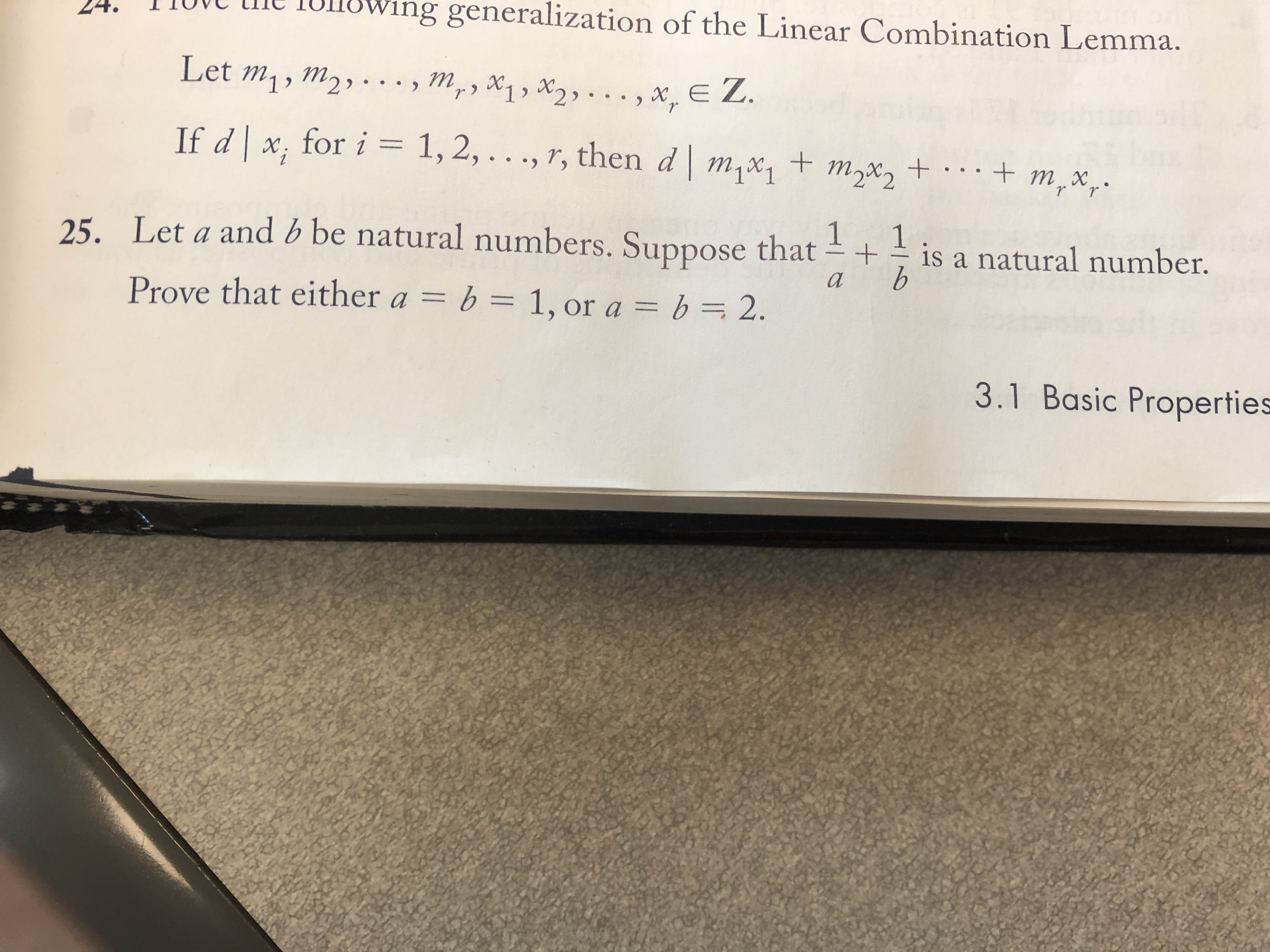# 24. TIOieing generalization of the Linear Combination Lemma.Let mi, m2If d x, for i 1,2,. . ., 7, then d m%2mxmy x1 , 2) .. x, EZis a natural number.25. Let a and b be natural numbers. Suppose thatProve that either ab 1,or a b 2.3.1 Basic Properties

Question
5 viewshelp_outlineImage Transcriptionclose24. TIO ieing generalization of the Linear Combination Lemma. Let mi, m2 If d x, for i 1,2,. . ., 7, then d m%2mx my x1 , 2) .. x, EZ is a natural number. 25. Let a and b be natural numbers. Suppose that Prove that either ab 1,or a b 2. 3.1 Basic Properties fullscreen
check_circle

Step 1

The values of variables and expression given below are natural numbers.

Step 2

The expression can be written as,

Step 3

This expression is a natural number. So, the denominator should be a factor of nu...

### Want to see the full answer?

See Solution

#### Want to see this answer and more?

Solutions are written by subject experts who are available 24/7. Questions are typically answered within 1 hour.*

See Solution
*Response times may vary by subject and question.
Tagged in

### Math